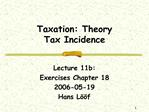DownloadDownload PresentationTaxation: Theory Tax Incidence

# Taxation: Theory Tax Incidence

Télécharger la présentation## Taxation: Theory Tax Incidence

- - - - - - - - - - - - - - - - - - - - - - - - - - - E N D - - - - - - - - - - - - - - - - - - - - - - - - - - -
##### Presentation Transcript

1. 1 Taxation: Theory Tax Incidence Lecture 11b: Exercises Chapter 18 2006-05-19 Hans L��f

2. 2 Outline 1. Tax incidence (burden) in Competitve markets 2. Tax incidence in Non-Perfect Competitve markets 3. Equivalent Taxes

3. 3 Main topics 1. What is ment by incidence of tax? Why is it that those who ultimately bear the burden of a tax may differ markedly from those upon whom the tax is legally imposed? 2. What determines who bears the burden of taxes? How does it depends on the elasticity of demand and supply? On whether markets are competitive or not. Why might it differ between the short run and the long run? 3. Why are some taxes that appear to be markedly different really equivalent?

4. 4 Important key concepts .

5. 5 Use the price/output diagram and/or mathematical expressions in order to explain [1.] * It makes no difference whether a tax is imposed on suppliers of a factor or a commodity or on the consumers. Instead, who bears the burden of the tax depends on the demand and supply elasticities, and on wheter the market is competitive or noncompetitive. Taxes induce changes in the relative prices, and it is this market response that determines who bears the tax

6. 6 Definition: elasticity of demand The elasticty of demand gives the percentage change in quantity as a resultat of a 1 percent change in price h= (DQ/Q) / (Dp/p)

7. 7 Definition Definition of Price Elasticity of Demand: Price elasticity of demand is defined as the percentage change in quantity demanded for a unit percentage change in the price of the good. So Price elasticity = ed = (percentage change in quantity demanded) / (percentage change in price) Note: Due to the law of demand, if %change in price is positive, %change in quantity must be negative and vice versa. So price elasticity of demand is always negative. So in most discussions the negative sign is ignored. Since elasticity is the ratio of two percentage changes, it is unit-less. Elasticity can be greater than, less than, or equal to 1. If ed > 1, demand is elastic in that price range. If ed < 1, demand is inelastic in that price range. If ed = 1, demand is unit-elastic in that price range. If ed is almost 0, demand is perfectly inelastic. If ed tends to infinity, demand is perfectly elastic

8. 8 Definition: elasticity of supply The elasticty of supply gives the percentage change in quantity as a resultat of a 1 percent change in price h= (DQ/Q) / (Dp/p)

9. 9 Definition Elasticity of Supply: Like demand, price elasticity of supply is the % change in quantity supplied divided by the percentage change in price of the good. Similar to demand, we can define elastic, inelastic, and unit elastic supply. A vertical supply curve is perfectly inelastic while a horizontal supply curve is perfectly elastic. Elasticity of supply depends on: Long run versus short run - Producers can adjust production more in the long run than in the short run. So elasticity of supply increases in the long run. Expected future prices - when production takes time, quantity supplied is more responsive to expected future price changes than to current price changes

10. 10 Use the price/output diagram and/or mathematical expressions in order to explain [2.] * In a competitive market, if the supply is completely inelastic or of demand is completely elastic, the tax i borne by PRODUCERS. If the supply is completely elastic or demand is completely inelastic, the tax in entirely borne by CONSUMERS.

11. 11 Use the price/output diagram and/or mathematical expressions in order to explain [3.] * A tax on a monopolist may be shifted more than 100 percent. That is, the price by consumers may rise more than the tax.

12. 12 Use the price/output diagram and/or mathematical expressions in order to explain [4.] * The general equlibrium incidence of tax, taking into account repercussions/effects in all industries, may differ from the partical equlibrium incidence The incidence of a tax my be different in the long run than in the short run.

13. 13 Use the price/output diagram and/or mathematical expressions in order to explain: [5.] * A tax on output (A uniform sales tax), a proportional income tax, and a uniform value added tax, are equivalent. A uniform tax on wages and uniform tax on consumption are equivalent.